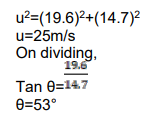Deepak Scored 45->99%ile with Bounce Back Crack Course. You can do it too!

# A person is standing on a truck moving with a constant velocity

Question:

A person is standing on a truck moving with a constant velocity of $14.7 \mathrm{~m} / \mathrm{s}$ on a horizontal road. The man throws a ball in such a way that it returns to the truck after the truck has moved $58.8 \mathrm{~m}$. Find the speed and the angle of projection

(a) as seen from the truck,

(b) as seen from the road.

Solution:

Time taken by ball=Time taken by truck to cover $58.8 \mathrm{~m}$

Time $=\frac{\text { distance }}{\text { speed }}=\frac{58.8}{14.7}=4 \mathrm{sec}$

(a)

For Truck, ball will seems to travel in vertical direction

Time to reach $\mathrm{H}_{\max }$ by ball= $=\frac{\overline{2}}{2}=2 \mathrm{sec}$

$v=0 ; a=-g ; t=2 \mathrm{sec}$

$\mathrm{v}=\mathrm{u}+\mathrm{at}$

$0=\mathrm{u}-\mathrm{g} \times 2$

$\mathrm{u}=19.6 \mathrm{~m} / \mathrm{s}$ (upward direction)

(b)

From road, motion of ball will be projective

Now, usin $\theta=19.6$

$U \cos \theta=14.7$